# The ACCU 2011 Crypto Challenge

The ACCU 2011 Crypto Challenge

By Richard Harris

Ready for the biggest challenge yet? Richard Harris throws down the gauntlet.

For a third time I have had the pleasure of contributing a cryptographic puzzle to the fund raising efforts for Bletchley Park during the ACCU conference.

You may recall that the first two puzzles reflected the development of modern cryptosystems, beginning with electro-mechanical rotary ciphers such as the Enigma machine and followed by electronic stream ciphers such as RC4, and were designed to so that they could be broken with pencil and paper alone if their weaknesses were spotted.

As with the previous two puzzles a bonus question was included that was not possible to answer if the puzzle was solved by brute force rather than analysis.

So that you too might have a go at it, I present the puzzle below followed by its historical inspiration, its solution and the names of the conference delegates who cracked it.

## The challenge

### Encoding

The enemy are using a 32 character alphabet encoded as 5 bit signed binary numbers ranging from -16 to +15. The # character is a control code indicating that the following character should be interpreted as its numerical value rather than as a letter or punctuation. The full table of character mappings is given below.

```  ---------------- +++++++++++++++
11111110000000000000000000111111
65432109876543210123456789012345
#abcdefghijklmnopqrstuvwxyz ?!.,```

### Encryption

The enemy are using a public key cryptosystem in which the private key is a set of variables containing the numeric encodings of randomly chosen characters.

The public key is a set of randomly generated polynomials in those variables, all of which, when calculated with the values from the private key, yield zero.

To transmit a message character, they first generate a new random polynomial for each of those in the public key. They multiply each pair and add the products together to create an encryption polynomial that must also yield zero when evaluated with the private key.

They finally add the encoded message character to yield the ciphertext polynomial.

To decrypt the ciphertext polynomial they simply evaluate it with the values in the private key; since the encryption polynomial will have a value of zero, the ciphertext polynomial will have a value of the encoded message character.

### Example

The characters ‘r’ and ‘s’ give the private key variables x and y values of 2 and 3 respectively. The randomly generated polynomials x 2 y + y and xy 2 yield 15 and 18 respectively and hence we can choose the polynomials x 2 y + y -15 and xy 2 -18 as the public key.

Multiplying these by the randomly generated polynomials y +1 and x -1 respectively and summing yields an encryption polynomial of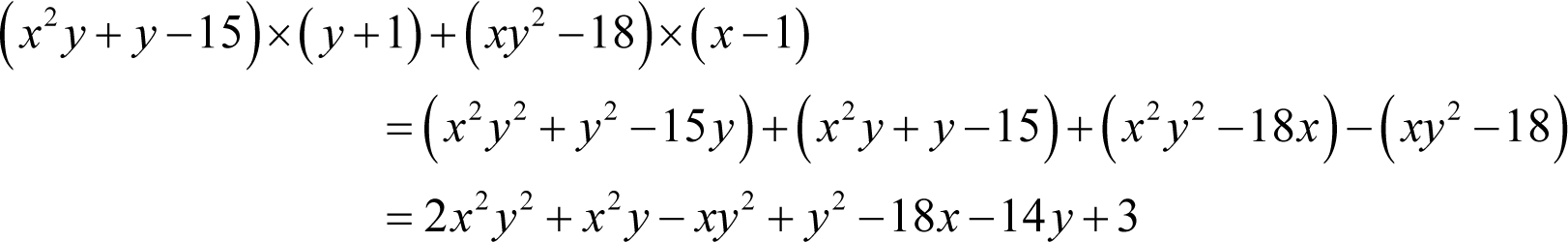The character ‘l’ can be encrypted by adding -4 to yield a ciphertext polynomial of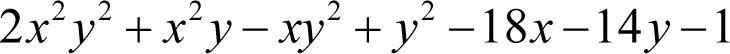Evaluating this ciphertext polynomial with the private key values for x and y reveals the message.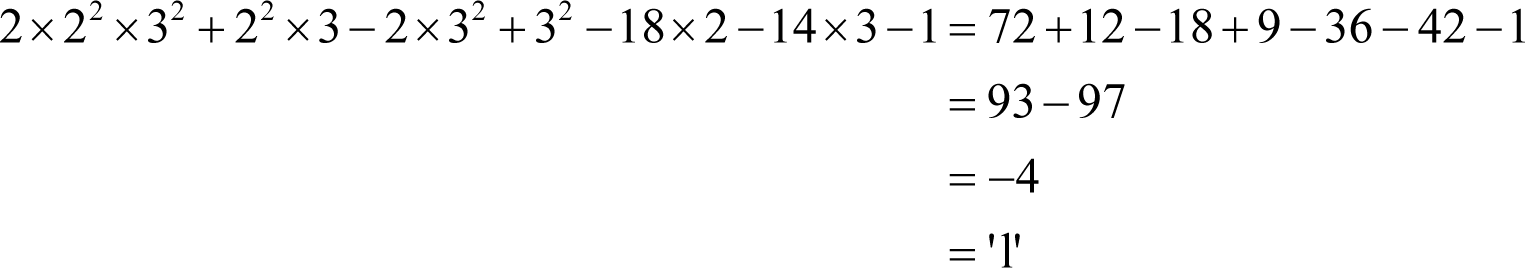### Public key

The enemy’s public key equations are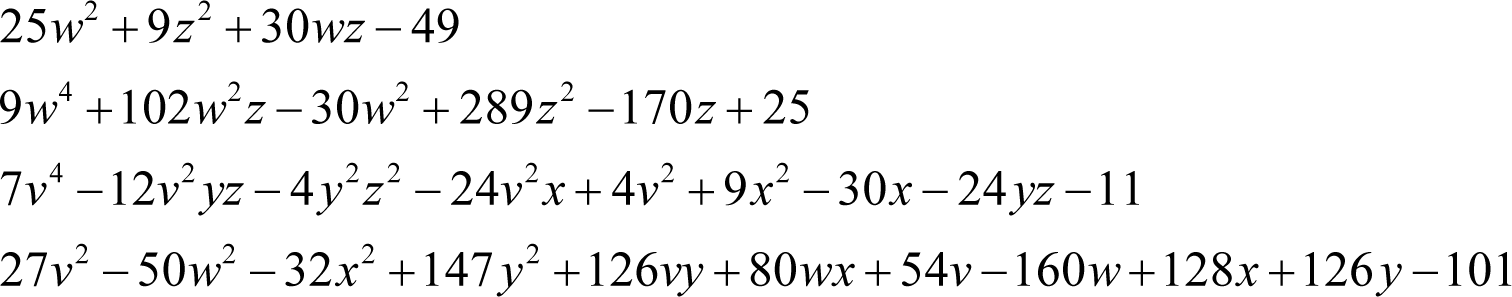### Bonus question

Assuming the worst possible ordering that is logically consistent with a cryptanalysis, what is the minimum necessary number of trial values that must be fed into these public key equations, as opposed to known values derived from them, to ensure that we can successfully recover the enemy’s private key values?

## The historical justification

Historically, one of the biggest problems in cryptography was exchanging the secret keys needed to encrypt and decrypt messages.

For example, the development of effective electronic ciphers required the exchange of sequences of random numbers. One way to do this was to hire a courier to carry a tape containing such a sequence between one party and another, perhaps in an aluminium briefcase handcuffed to his wrist as seen in so many spy movies.

The problem was then how to securely exchange the keys to the briefcase.

A solution is for the first party to courier an unlocked briefcase to the second party without the key. The latter can put their tape into it, close and push a button to lock it, and then send the courier back to the first party to securely exchange their electronic key without ever having seen the briefcase key.

Of course, both parties must trust the courier…

This is essentially the idea behind public key cryptography; the first party provides a means for the second to hide a secret without providing the means to reveal it.

We do this by means of a trap door function, that is to say a function that is easy to compute but difficult to invert.

The canonical example is the factorisation of integers. It is easy to calculate the product of a pair of integer factors, but it is very much more difficult to determine from the product what those factors are.

Whilst this is certainly an interesting property of the integers, it is not at all clear on the face of it how it is of any use.

The trick is to construct a function which depends upon the product and is difficult to invert unless you know its factors.

The RSA cryptosystem is an example of just such a function.

The first step is to choose a pair of prime numbers p and q and compute their product n . For example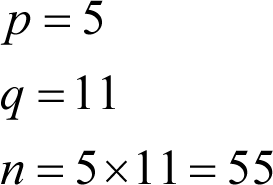Next we calculate the product of p -1 and q -1, denoted by ϕ( n )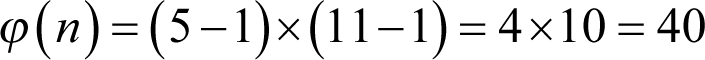Now we must choose a value e that is both smaller than ϕ( n ) and has no common factors with it. In our example ϕ( n ) has prime factors of 2 and 5, so we may choose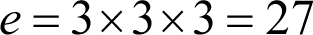Next we must calculate the value d which when multiplied by e yields a product that is one plus some integer multiple of ϕ( n )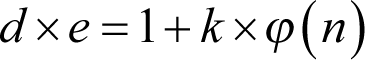Mathematically, this the multiplicative inverse of e under arithmetic modulo ϕ( n ) which we can write as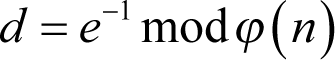We can do this using the extended Euclidean algorithm which we shall not cover here. Note that this inverse is guaranteed to be unique because e and ϕ( n ) have no common factors and in our example must be equal to 3.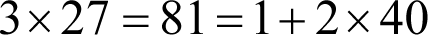The public key is comprised of the pair of integers n and e and the private key of the single integer d .

To encrypt a message m that has a value between 0 and n we calculate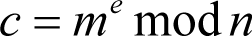For example, we shall choose 2 for our message m . The ciphertext c is consequently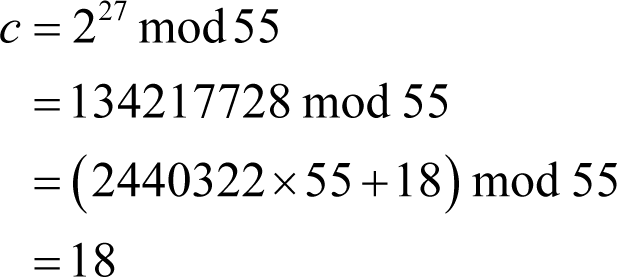To decrypt the ciphertext we compute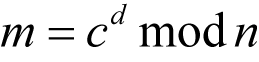In our example we have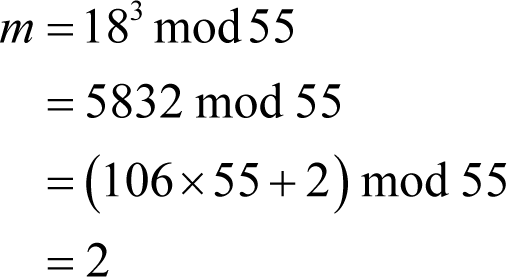and as if by magic we have recovered the message!

What makes RSA secure is that the private key d is the multiplicative inverse of the public key value e modulo ϕ( n ) which in turn depends upon the hard to find prime factors of the public key value n .

We can demonstrate why RSA works from Euler’s theorem which states that if m and n have no common factors, or in this case that the message is not equal to either of the prime factors of n , then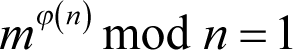Consequently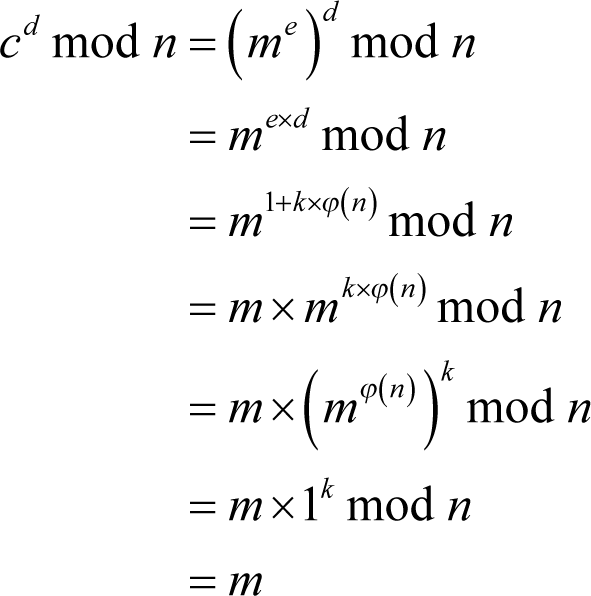since m is smaller than n .

The requirement that the message not be equal to either of the prime factors is not particularly burdensome in practice since they will be very, very large.

Now, our cryptosystem uses the difficulty in factoring integer polynomials rather than integers as its trap-door function. In the worked example we saw that it was relatively easy to construct a ciphertext polynomial from the public key polynomials.

That it is very much more difficult to reverse this process can be seen if you try to extract the message using just the ciphertext and public key polynomials.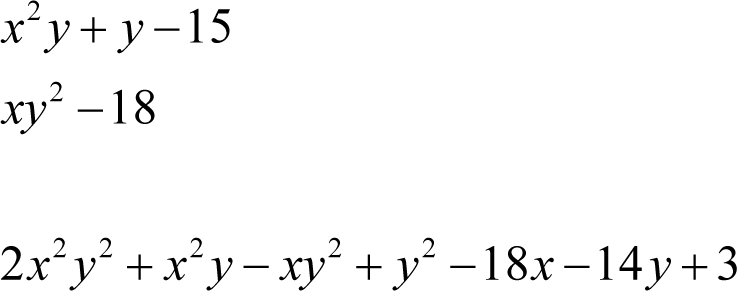Integer polynomials like these are known as Diophantine equations and are notoriously difficult to reason about.

Perhaps the most famous example is Fermat’s Last Theorem which states that the formula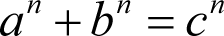has no solutions with integer a , b and c if n is greater than two.

Despite Fermat’s claim to have a proof that was too large to fit in the margin of the text in which he wrote his conjecture, it took over 350 years before the theorem was finally, and famously, proven by Andrew Wiles.

## The solution

In this challenge the ciphertext polynomial is not given, so it is ‘simply’ an exercise in finding the roots of the non-linear public key Diophantine equations; those values of the private key variables for which they all equate to zero.

Brace yourself…

To begin, consider the first public key equation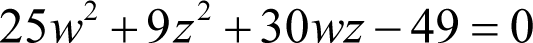Three of these terms are suspiciously perfect squares, 25 w 2, 9 z 2 and 49, and if we factorise the terms we have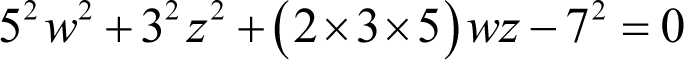The first three terms are those we should expect from the square of a sum of two terms and the last is the negation of a perfect square. Expressing the first three as just such a square and moving the last over to the right hand side yields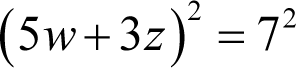We can now take the square roots of both sides of the equation to reveal two possible relationships between w and z .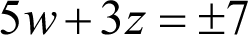The analysis of this first equation is a significant clue as to how we should proceed; the entire puzzle is in fact an exercise in solving quadratic equations and the key to solving it is realising this.

Examining the second equation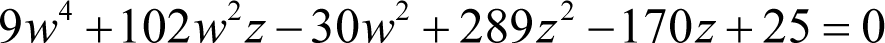we should note that once again we have three perfect squares in the terms 9 w 4, 289 z 2 and 25.

Factorising all of the terms yields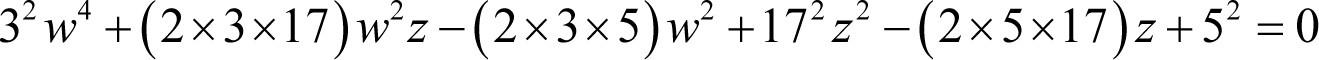This time we have three non-squares that just happen to be twice the product of two of the three squared terms. Noting that it is only the terms with a single factor of five that are negative, we can express this equation as the square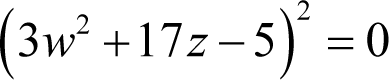and hence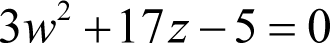We now have two possible pairs of simultaneous equations in w and z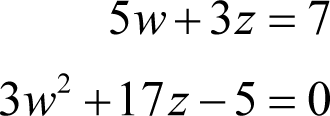and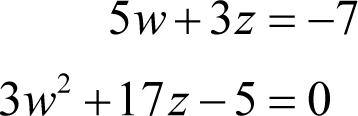and we are consequently in a position to examine the possible values of those private key variables.

Beginning with the first, we have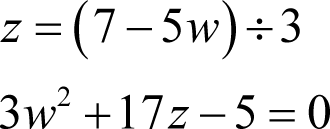Multiplying the second equation by three, substituting z from the first and simplifying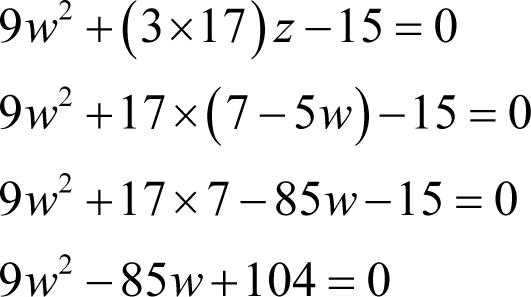At this point we can apply the rule for solving quadratic equations that we learnt in secondary school; minus a plus or minus the square root of b squared minus four times a times c all divided by two times a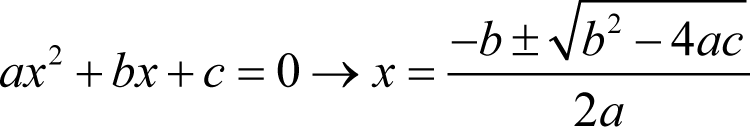In this case this means that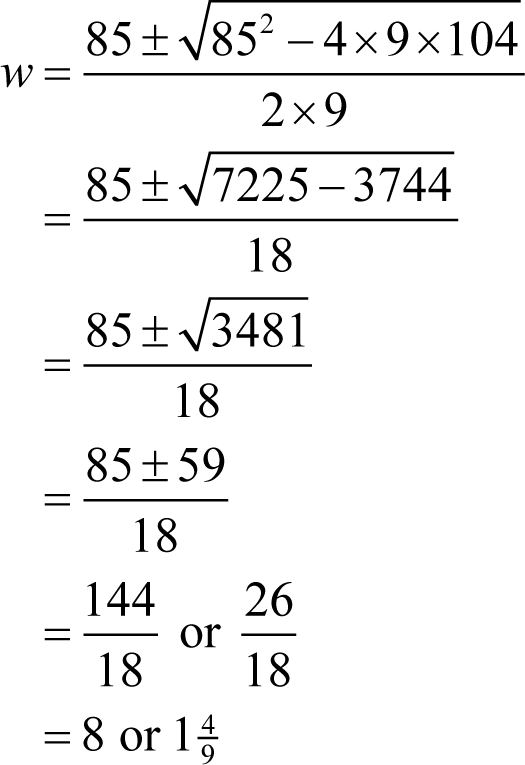We therefore have one candidate value for w of eight, but we must solve the second pair of equations to be sure that it is unique. Rearranging the first of those equations yields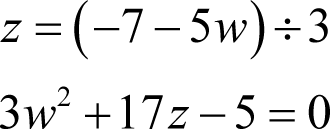Following the same approach as before we have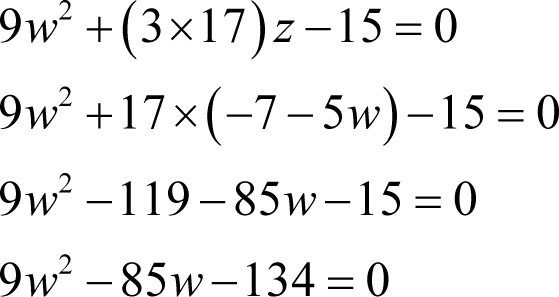Applying the rule for solving quadratic equations yields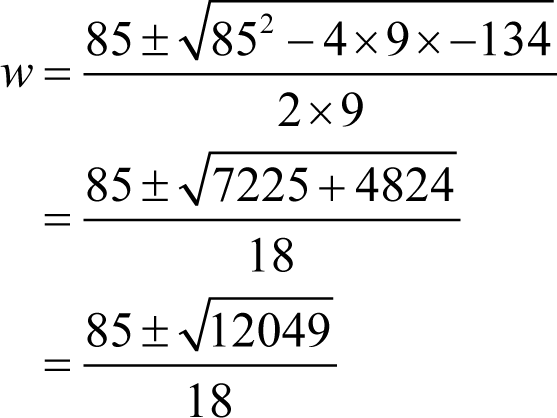We must stop here because 12049 is a prime and hence this equation cannot result in a valid value for w .

With the unique valid value we have for w we can now calculate the correct value for z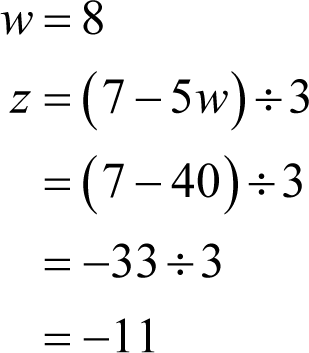Having solved the first two public key equations we are ready to analyse the third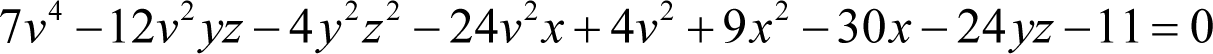Ignoring the coefficients for now, this equation has a constant term, terms in v 2 , x and yz , their squares and terms in all of their pair products except xyz . The fact that this term is missing mean that this cannot be a square of a sum of terms in v 2 , x and yz .

It is, however, perfectly consistent with a sum of squares of the form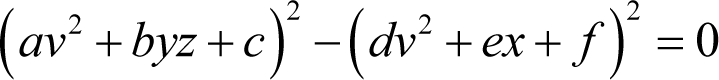Noting that the v 4 , v 4 and constant terms must be split between both squares, we can group the related terms together on either side of the equals sign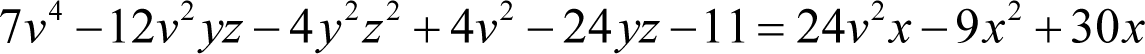We can see immediately that the y 2 z 2 and x 2 terms on the left and right hand side of the equation have negative coefficients. We must therefore negate both sides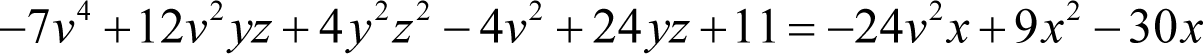Now we must add the terms that are missing on the right hand side of the equation to both sides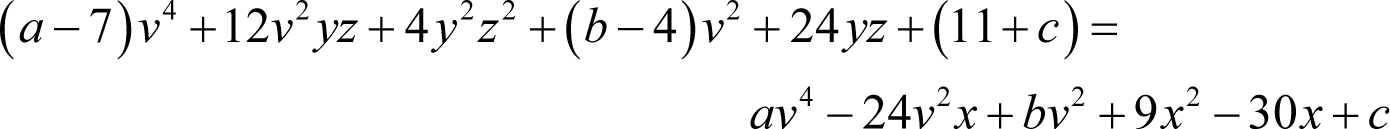Now, if both sides are squares of sums, these unknown coefficients must conform to the very specific relationships this implies.

Firstly, the constant c must be related to the x and the x 2 coefficients by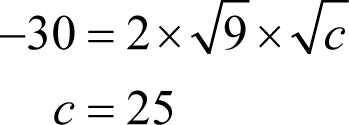giving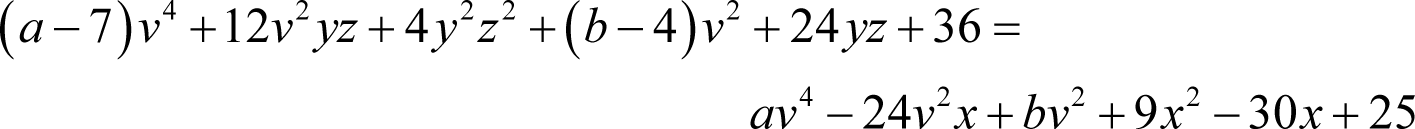reassuringly making the constants on both sides of the equation perfect squares.

Similarly, the coefficient of v 4 on the right hand side, a , must be related to the coefficients of v 2 x and x 2 by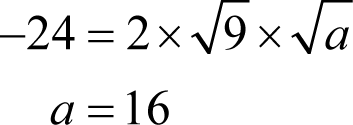giving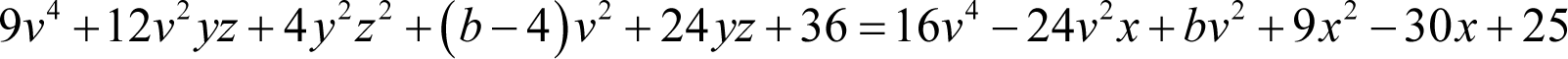once again yielding perfect squares exactly where we want them!

Finally, relating the v 2 coefficient on the right hand side, b , to those of v 4 and the constant means that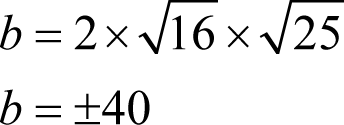Given that the coefficients of both x and v 2 x are negative, b must be positive giving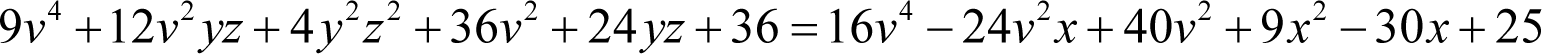Partially factoring the coefficients yields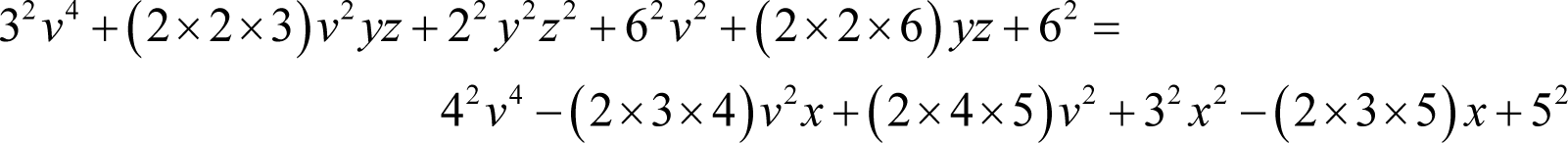so we can express this as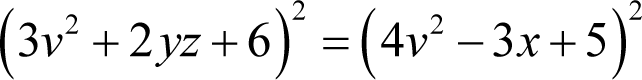and consequently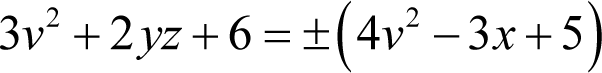Now we have already determined that z is equal to minus 11, so we can substitute it into this equation to yield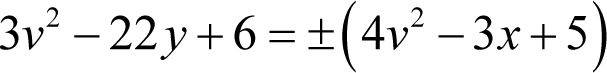Now we are on the home stretch with just one public key equation left to consider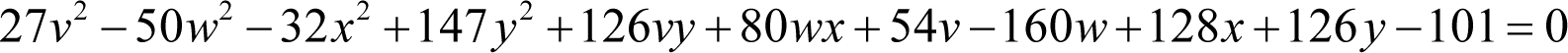Leaving aside the coefficients once again, it is clear that this formula is also formed from two quadratic equations, one in v and y , the other in w and x , with the constant term split between them.

Rearranging gives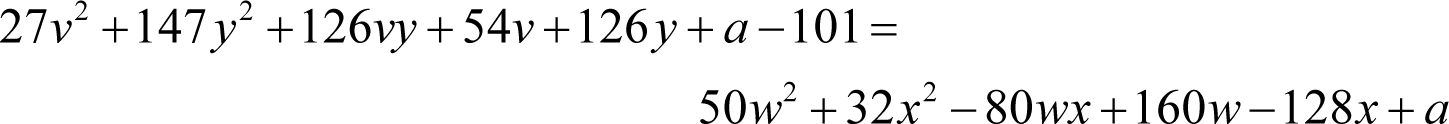A problem immediately presents itself in that the coefficients of the squared variables are not squares.

To address this we must partially factor the coefficients first .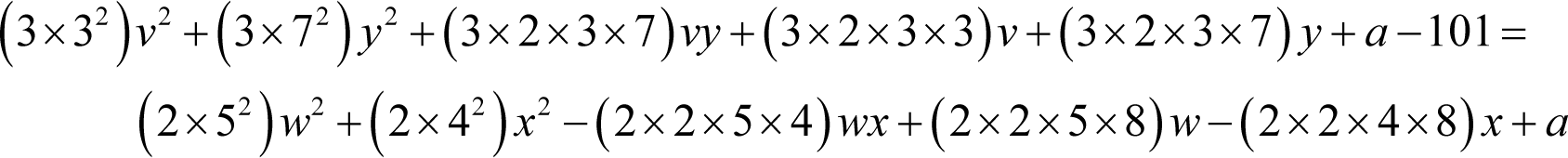So far, this is consistent with the left hand side being three times a square of a sum and the right hand side being twice the square of a sum.

If we take these factors out, we shall see whether it gives a consistent value for the constant a .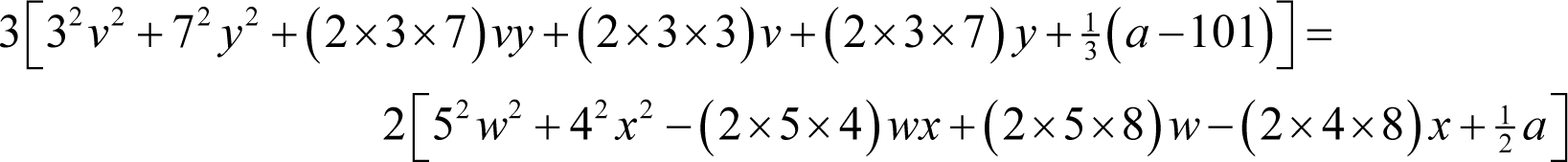From the right hand side, following a similar argument that we used for the previous formula, if it is a square then a must satisfy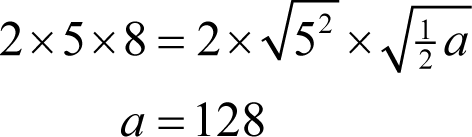and therefore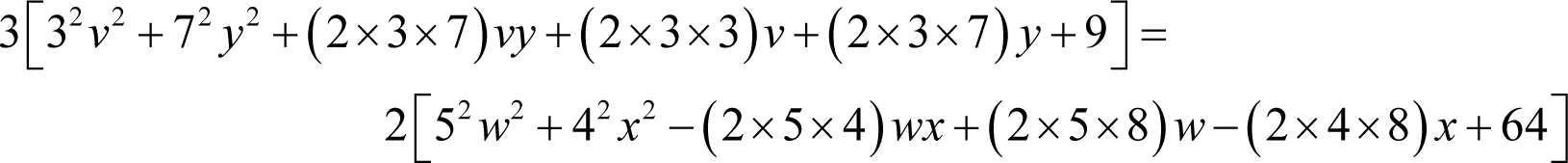giving us exactly the squares we seek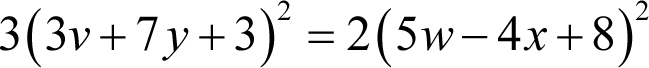Given that the private key variables are integers and that the square root of three is not an integer multiple of that of two, this equation can only hold when both the left and right hand sides are equal to zero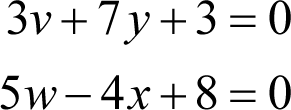We already know that w is eight, so from the second equation we have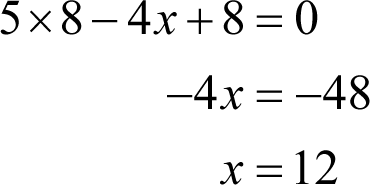Having found x we can further simplify the equation we derived from the third public key equation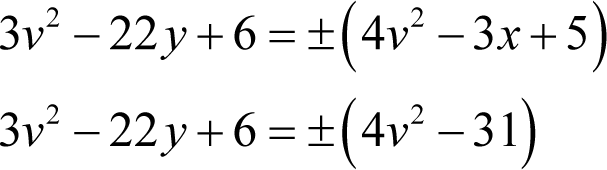From the first of the equations we derived from the fourth we can relate v and y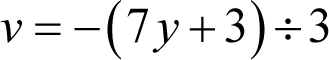Substituting this into the above is more easily achieved if we multiply the latter by nine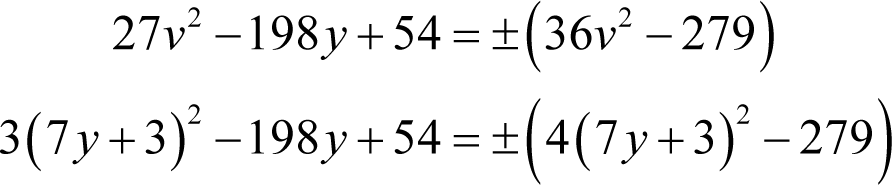Expanding the squares yields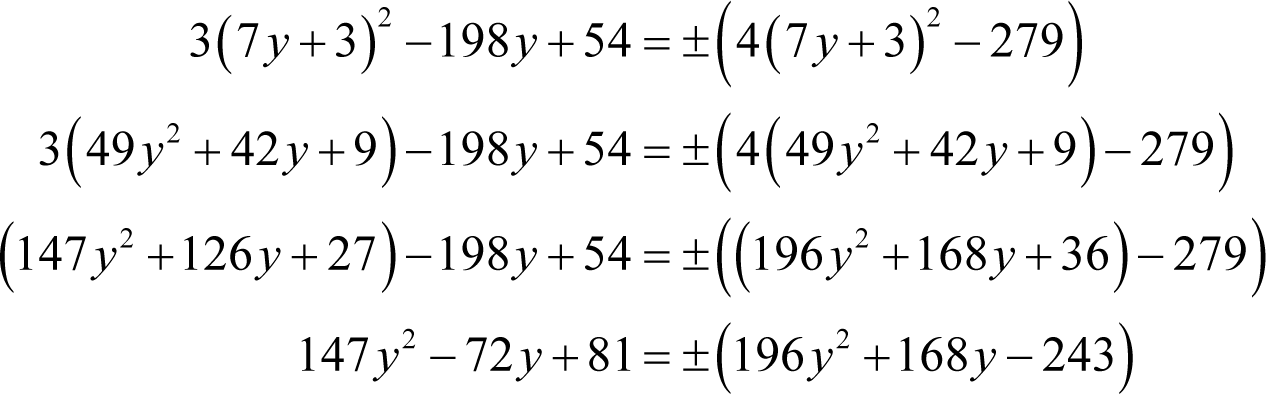Once again we have two possibilities to consider, both of which yield simple quadratic equations.

Firstly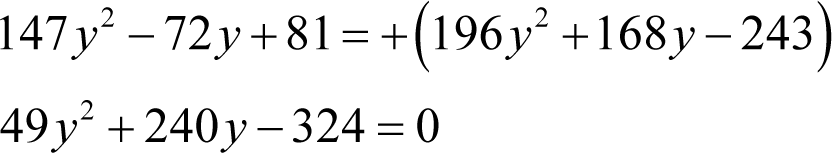Using our trusty quadratic equation rule again we have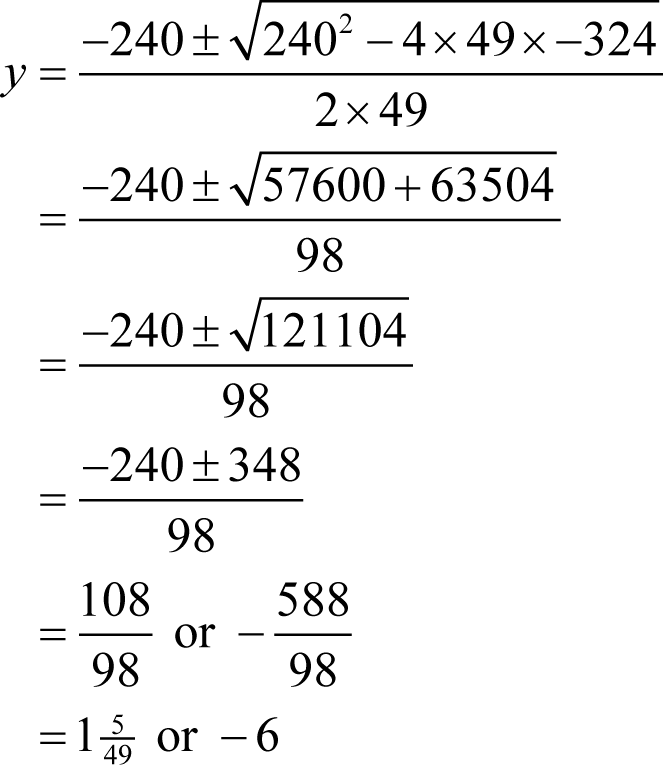giving us a candidate value for y .

Of course we must check the second possibility to be sure we have the correct value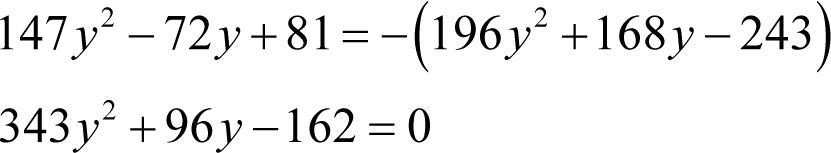Solving for y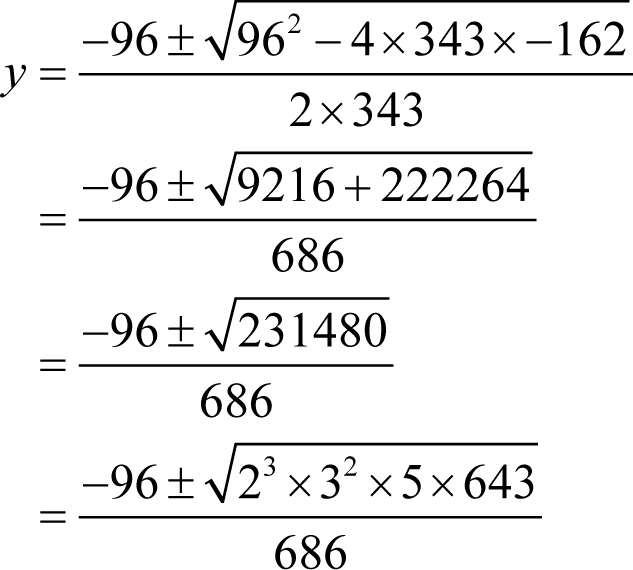Consequently y must be equal to minus six and thus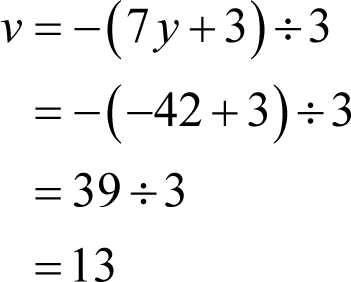giving us the values of all of the private key variables without our having to guess at any of them!

## An (insincere) apology

Now I recognise that this puzzle was somewhat trickier than the previous puzzles and for that I offer my apologies. In my defence, it was quite difficult designing a public key crypto challenge that satisfied my own requirements; those of novelty and a pencil and paper solution. Everything else I considered was either trivially solved using brute force or impossible to solve using pencil and paper.

And after all, it was an exercise in public key cryptography; of course it was going to be difficult!

I’m afraid that this has been the last of the crypto challenges; the next logical step would have been a puzzle based on quantum cryptography but I am reasonably certain that it is quite beyond my meagre faculties to design such a beast.

## And finally…

Congratulations are due to Gary Duke who successfully cracked the Crypto Challenge and to Per Liboriussen who was just one step from doing so.

I should also like to thank everyone who took part and everyone who donated to Bletchley Park.